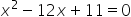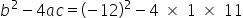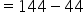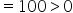Mathematics
Easy

Question

# Find the number of solutions for the equation.

## 0    1    2    Either 1 or 2Hint:

## The correct answer is: 2

### Step 1 of 1:We have given an equation.Now,, so, it has two real solutions

The discriminant is the part of the quadratic formula found within the square root.

### Related Questions to study#### With Turito Foundation.#### Get an Expert Advice From Turito.